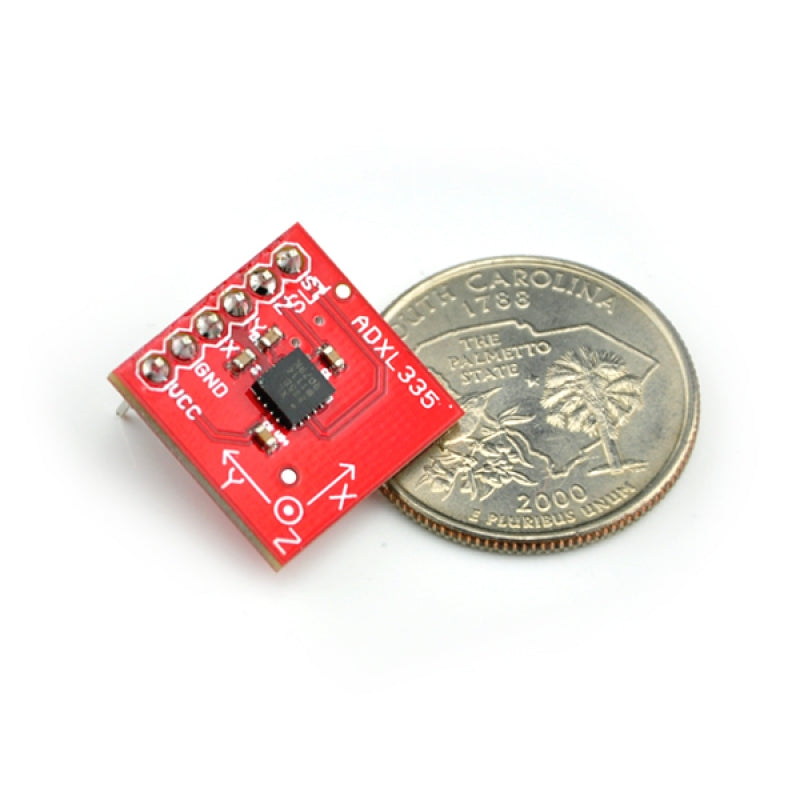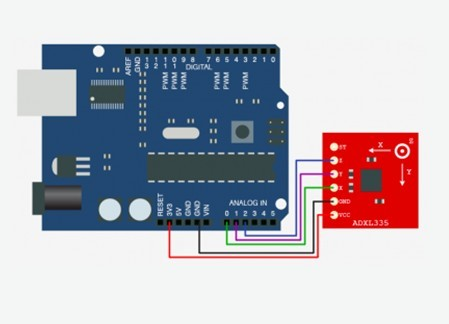Tinysine

G4F2B4369A037B

100 In Stock

Regular price US\$3.95
###### Shipping Fees are displayed on the cart page.

This is the latest in a long, proven line of analog sensors - the holy grail of accelerometers. The ADXL335 is a triple axis accelerometer with extremely low noise and power consumption - only 320uA! The sensor has a full sensing range of +/-3g.

There is no on-board regulation, provided power should be between 1.8 and 3.6VDC.

Dimensions: 0.7x0.7"

## Hooking it Up:

Here is the guide that illustrates how to connect an Arduino to the ADXL345 breakout board. The following is a table describing which pins on the Arduino should be connected to the pins on the accelerometer:

 Arduino Pin ADXL345 Pin 0 Z 1 Y 2 X 3V3 VCC Gnd GNDTest Code:

const int xPin = 0;
const int yPin = 1;
const int zPin = 2;

//The minimum and maximum values that came from
//the accelerometer while standing still
//You very well may need to change these
int minVal =270;
int maxVal =440;

//to hold the caculated values
double x;
double y;
double z;

void setup(){
Serial.begin(9600);
}

void loop(){

//read the analog values from the accelerometer

//convert read values to degrees -90 to 90 - Needed for atan2
int xAng = map(xRead, minVal, maxVal, -90, 90);
int yAng = map(yRead, minVal, maxVal, -90, 90);
int zAng = map(zRead, minVal, maxVal, -90, 90);

//Caculate 360deg values like so: atan2(-yAng, -zAng)
//atan2 outputs the value of -π to π (radians)
//We are then converting the radians to degrees
x = RAD_TO_DEG * (atan2(-yAng, -zAng) + PI);
y = RAD_TO_DEG * (atan2(-xAng, -zAng) + PI);
z = RAD_TO_DEG * (atan2(-yAng, -xAng) + PI);

//Output the caculations
Serial.print("x: ");
Serial.print(x);
Serial.print(" | y: ");
Serial.print(y);
Serial.print(" | z: ");
Serial.println(z);

delay(100);//just here to slow down the serial output - Easier to read
}